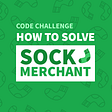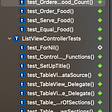# Combining Operators In RxSwift

In this article I will be covering 7 types of combining operators in RxSwift.

• StartWith
• Concat
• Merge
• CombineLatest
• With Latest Form
• Reduce
• Scan

StartWith:

Using this operator you can pass the desired value to the beginning of a sequence. Assume that I’ve a sequence of 2,3,4 if I apply the startWith operator and pass in a value “1” I will endup with the following sequence 1,2,3,4. Lets have a look at marble diagram for better understanding.

This operator is not limited to Int only you can use to any kind of data type. Lets have a look at practical implementation.

As you can see in the image above our sequence was containing two elements “Hello” & “World” but later on we applied the startWith operator passing a value “Hey” and the print statement shows “Hey” was added in the beggining of sequence.

Concat:

This operator simply join two observables.

The code above simply shows us the firstObservable and secondObservable combined using Observable.concat(). Note that the new observable sequence is something like this [Hello, world, hey, hi] since the second observable was passed after firstObservable on line 12 thats why the values of secondObservable is at the end of concatenated Observable. If I pass on line 12 [secondObservable,firstObservable] then the concatenated sequence will look like this [hey, hi, hello, world].

Merge:

Unlike the concat operator who concat sequences based on the order they are passed in the merge operator merges the values of sequence based on their arrival. Confusing? let’s have a look at marble diagram.

As you can see the marble diagram when we applied the merge operator regardless the order of how we passed in the observables the merge operator only care about the order of arrival for each individual element. Let’s have a look at practical implementation.

The print statement in above image proves us that merge operator only care about the order of elements and not the order of its parameters.

CombineLatest:

This operator will always keep track of the latest values coming from each sequence . Let’s have a look at marble diagram.

The image above shows three sequences the top two are being passed in combine operator and it is returning latest values from both of the sequences simultaneously.

The top sequence is ‘1,2,3,4’ and bottom sequence is ‘5,5,5,6,7,7’. When 1 is received the bottom sequence received 5 and when 2 is received from top sequence we still didn’t get any new value from bottom sequence hence the latest values remains to be 5. This is how this operator will continue.

Lets have a look at practical implementation.

As you can see in the image above two values are being printed at the same time one from the firstObsservable and second from the secondObservable i.e top and bottom sequences in marble diagram respectively.

WithLatestFrom:

Assume that we have a button and a textfield both are observable button we only want to get the value of textfield when the button is tapped. In this kind of scenario withLatestFrom comes in handy. By using withLatestFrom when ever I tap the button I get the latest value from the textfield for better understanding I’ve create a marble diagram.

Ignore the redline under textField 😂 ppt won’t understand camel casing. as you can see in the diagram during our first tap the latest value we had from textfield was “Pakis” so with this tap we get “Pakis” as value and during our second tap the latest value of textfield was “Pakistan” and we got that value.

Here is a practical implementation of withLatestFrom.

I don’t think so i need to explain the practical implementation after the marble diagram but you can compare the print statement from the image above with the result in marble diagram.

Reduced:

Applies a function to each item of observable sequence and returns a final value.

In the above image we have three things to consider “Seed”, “X” & “newValue”. So what this function is basically doing and what are these three values. The seed is the initial value lets say seed = 0 . As we know the reduce method applies function to each item it means its using some king of loop hence x = “item of observable sequence” which means at first its 1 then 2 then 3 and then 4 how ever the ‘newValue’ is the stored values of pervious result we are returning ‘x+ newValue’ when x is 1 newValue = seed and when x is 2 newValue = newValue + x (when x was 1). When x is 3 newValue = newValue + x(when x was 2) and so on. The final value we get when seed is 0 should be 10.

`seed = 0 x = 1 newValue = 0return 1 + 0 seed = 0 x = 2newValue = 1return 2 + 1seed = 0 x = 3newValue = 3return 3 + 3seed = 0 x = 4newValue = 6return 6 + 4no more items in observable sequence hence final value = 10`

if we set seed = 10 in above scenario then the final value would be 20.

Scan:

It is very similar to .reduce() the only difference is reduce gives you a final value however scan will provide you a sequence with newValue as items in it. When mean after applying function to each item it adds the newValue to the final observable.

`seed = 0 x = 1 newValue = 0return 1 + 01 + 0 added to final Observableseed = 0 x = 2newValue = 1return 2 + 12 + 1 added to final Observableseed = 0 x = 3newValue = 3return 3 + 33 + 3 added to final Observableseed = 0 x = 4newValue = 6return 6 + 46 + 4 added to final Observableno more items in observable sequence hence final value = 1,3,6,10`

I hope I was able to explain all of them briefly if you do like my article kindly follow me this encourages me to write more of the content about Swift.

--

--

--

## More from Umair Ishrat Khan

An energetic and motivated individual IOS developer/ Data Science Practitioner. Apart from computer science Martial arts interests me the most.

Love podcasts or audiobooks? Learn on the go with our new app.

## Eye-catching moments during 13 hours of development with Angular.## How to structure a React project## An enterprise approach to the Smart and Dumb components pattern in Angular## Promise-Based Detection of Element Injection## Chat-app Creation Using Vue.js, Nuxt.js, Node.js, Socket.IO, Vue-Socket.IO, Vuetify.js Technologies## How To Solve The Sock Merchant Challenge## Deploy a React Project on Nginx webserver to GCP VM Instance## What Makes a Successful Software Engineer?## Umair Ishrat Khan

An energetic and motivated individual IOS developer/ Data Science Practitioner. Apart from computer science Martial arts interests me the most.

## RxSwift usage with MVVM pattern.## Test Driven Development In iOS Swift Sample## AppDelegate Lifecycle 🥚🐣🐥🐓## Dynamic Table View Cell!